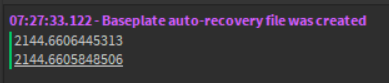# How does Roblox calculate pi?

Hello this is my first post.

I am building a simulation in gravity mechanics. Mass of an object is important to the math required to model this simulation. Mass in Roblox is the volume of the part I’m measuring. In order to proof this, and the relationship CustomPhysicalProperties.Density has with mass, I wrote a quick script to test this.

I compare Part:GetMass() with my own calculation which uses math.pi. The results are extremely close. However the output shows a very slight difference numerically. I have tired to mess around with pi and how many decimal places I’m using, or even rounding up to. But I am unable to make a perfect match.

How does Roblox physics engine calculate pi? How many decimals does it use? Any thoughts?
Here is my proof script and the output:So they don’t actually calculate it, they just store the value as a double floating point number IEEE 754. It is stored with 64 bits with the furthest left bit representing +/- the next 11 being the exponent and the final 53 bits being the fraction. Basically it allows for up to 17 significant digits to be stored.

5 Likes

Ok I think I understand better now. Thank you.